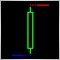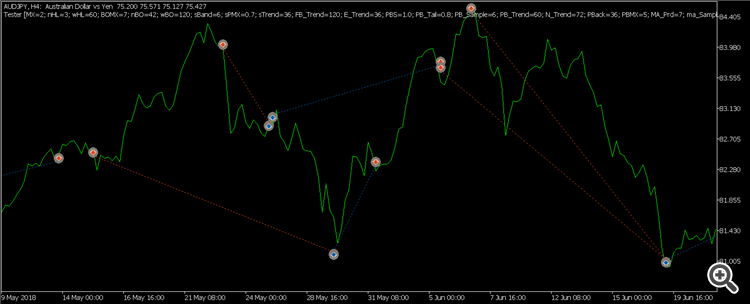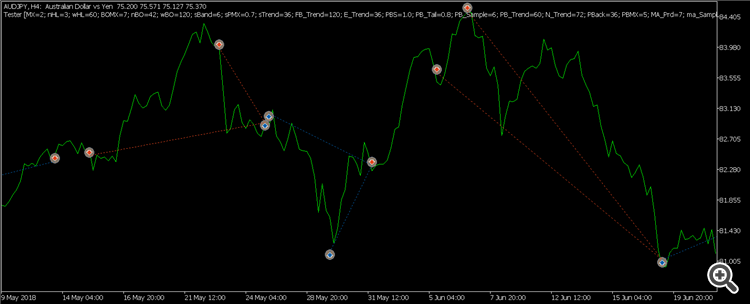# code behaves differently in every tick mode vs one minute OHLC5737every tick modeohlc mode

my code closes position by averaging. the codes behave different in every tick vs one minute ohlc mode

```void ClosePosition(ENUM_POSITION_TYPE postype)
{
//---

double   accprofit  = 0,
posprofit  = 0,
unitlots   = 0,
unitprofit = 0;

for(int i = PositionsTotal() - 1; i >= 0; i--)
{
if(position.SelectByIndex(i))
{
if(position.Symbol() == _Symbol && position.Magic() == MagicNum)
{
if(position.PositionType() == postype)
{
posprofit = position.Profit();

if(posprofit > 0 || accprofit + posprofit > 0)
{
accprofit += posprofit;
Sleep(800);
continue;
}

if(accprofit > 0)
{
unitprofit = MathAbs(posprofit) / (position.Volume() * 100);
unitlots   = MathFloor(accprofit / unitprofit) / 100;
unitlots   = NormalizeDouble(unitlots, 2);

if(unitlots >= min_vol)
{
Sleep(800);
break;
}
}
}
}
}
}

//---
}```

what is wrong?5737

not sure if the problem is due to requote

any idea how to set EA to trade in market execution mode?